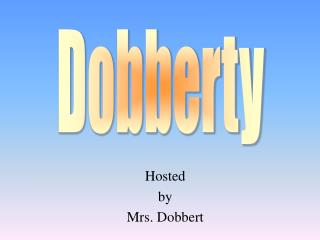DownloadDownload PresentationHosted by Mrs. Dobbert

# Hosted by Mrs. Dobbert

Télécharger la présentation## Hosted by Mrs. Dobbert

- - - - - - - - - - - - - - - - - - - - - - - - - - - E N D - - - - - - - - - - - - - - - - - - - - - - - - - - -
##### Presentation Transcript

1. Dobberty Hosted by Mrs. Dobbert

2. Vocabulary Calculator Calculations Dobbert’s Doozies 100 100 100 100 200 200 200 200 300 300 300 300 400 400 400 400 500 500 500 500

3. Row 1, Col 1 Residual is the distance between the data y value and the predicted y value. Deviation is the difference between the data y value and the average y value. y – “y hat” Describe the difference between residual and deviation. Cite appropriate notation.

4. 1,2 • Exponential Make a scatter plot of the data. State the type of function that models the data set. Be prepared to share with the class. X 1 2 3 6 7 8 9 10 Y 10643 4776 2194 637 483 371 298 222

5. 1,3 Power or exponential Make a scatter plot of the data. State the type of function that models the data set. Find the Regression equaiton. X 100 200 500 700 1000 Y 60 46 31 26 21

6. 1,4 Interpolation corresponds with a value within the domain of the data set. Extrapolation corresponds with a value outside the domain of the data set. Describe the difference between interpolation and extrapolation.

7. 2,1 R is the correlation coefficient and describes the trend of the data, positive or negative, strong or weak. R2 is the coefficient of determination and describes the percent of variability of the data accounted for by the regression equation. What information does r and r 2 provide us? What vocabulary term is associated with r and r 2 ?

8. 2,2 Y = 1.924x + 13.089 Find the appropriate regression equation for the set of data. X 10 14 16 20 25 26 30 Y 30 40 46 54 60 63 70

9. 2,3 792.833 Use lists on your calculator to find. SSdev Be prepared to share with the class. X 10 14 16 20 25 26 Y 30 40 46 54 60 63

10. 2,4 7.5 + 3.607lnx = y Algebraically find the logarithmic equation that contains the points (2,10) and (8,15).

11. 3,1 The centroid is the point defined by (x-average, y-average). It is the point that always lies on the linear regression equation. Define centroid. What is its significance with respect to a linear regression equation?

12. 3,2 Exponential Make a residual plot to determine if a linear or exponential equation better models the data set. X 0 2 4 6 8 10 Y .07 .2 .6 2 5 16

13. 3,3 0.990 Given the data set, calculate the coefficient of determination. Use the definition that includes SSres and SSdev. X 2 4 6 8 10 12 Y 10 17 21 28 35 38

15. 4,1 (Type the question for 4,1 here.)? Using sigma notation, write an Expression that express the sum of the square of the residuals.

16. 4,2 Yes…the points possess a random pattern. Based on the linear residual plot, is a linear model a good fit for the data? Support your claim.

17. 4,3 (Type the question for 4,3 here.)? Find the power and exponential regression equations. Paste the equations into y1 and y2. Create residual plots for the power and exponential models. Determine which model is a better fit. X 3 4 5 6 7 8 9 10 11 Y 46 41 36 31 27 24 21 18 15

18. 4,4 Tuesday, December 18 @ 10:20 State the date and time of the math Exam.

19. 5,1 R2 = (SSdev - SSres )/SSdev Write the expression that calculates The coefficient of determination.

20. 5,2 No, the plot indicated there is a function with a better fit. The plot has a non-random pattern. Based on the logarithmic residual plot, is a logarithmic model a good Fit for the data? Support your claim.

21. 5,3 Linear Find the linear and exponential regression equations. Paste the equations into y1 and y2. Create residual plots for the linear and exponential models. Determine which model is a better fit. X 3 4 5 6 7 8 9 10 11 Y 23 37 50 62 76 89 102 114 126

22. 5,4 L3 = (L2 – mean(L2))2 Data has been entered into lists 1 and 2, describe the rule to calculate State the rule for list 3 and any other Calculator instructions needed.